## Pages

### Function Composition

Here is where we introduce a new operation - composition of functions.  With this definition comes new notation.This new notation reads “f composed with g.”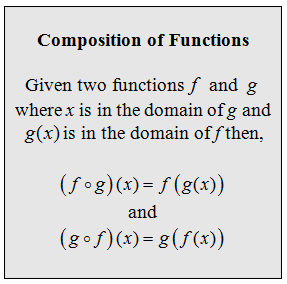The idea is to substitute one function into another function.

Given the functions f(x) = 5x – 4 and g(x) = 2x – 1.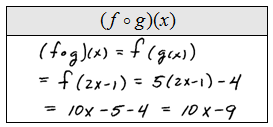Composition of functions is not necessarily a commutative operation, in other words, order matters.

Given the functions f(x) = x^2 – 9 and g(x) = x – 3.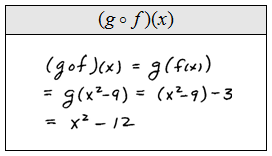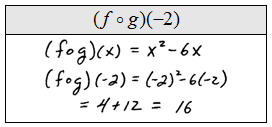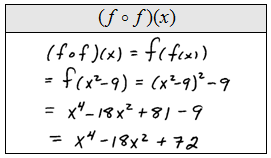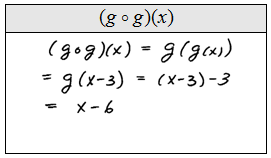Given the functions f(x) = x^2 + 1 and g(x) = sqrt(– 1) where ( x >= 1 ).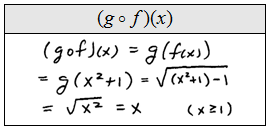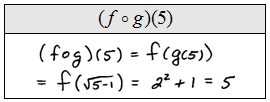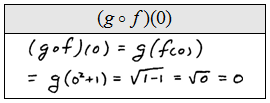At this point we must understand what happens to the domain of a composite function. In the above example it might appear that f o g has a domain of all real numbers.  In fact, the domain is restricted to [1, inf) because that is the domain of g.  The domain of f o consists of all the values in the domain of g that are also in the domain of f.

Given f and g find f o g and state its domain.